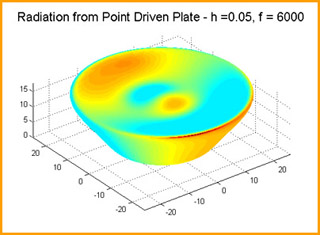MIT# [100% Off] INTRODUCTION TO NUMERICAL ANALYSIS FOR ENGINEERING (13.002J) Free Course Coupon

Updated: by ghatfanmerie

Click to get the course

8.0 hr
English
Free
Get the coupon in the end of description.

### Description

This course is offered to undergraduates and introduces students to the formulation, methodology, and techniques for numerical solution of engineering problems. Topics covered include: fundamental principles of digital computing and the implications for algorithm accuracy and stability, error propagation and stability, the solution of systems of linear equations, including direct and iterative techniques, roots of equations and systems of equations, numerical interpolation, differentiation and integration, fundamentals of finite-difference solutions to ordinary differential equations, and error and convergence analysis. The subject is taught the first half of the term.
If the coupon is not opening, disable Adblock, or try another browser.